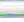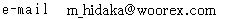## Theory of Relativity was Born Due to Mistakesin Deriving Equations

Mamoru Hidaka
Chofu city Tokyo Japan

The process of constructing new theoretical formulae involves expressing postulates in terms of functions followed by development of the resulting equations. Of the vast body of theoretical work reported to date, only the development of the theory of relativity omitted steps in this procedure because the basic mathematics defining the principle of the constant velocity of light were mental arithmetic, and therefore not recorded. The theory of relativity is a rare example of a theory that came to fruition as a result of mistakes in the mathematical process but which has had far reaching consequences in the field of physics and the modern history of mankind.

In the development of mathematical or physical theories, functions are used to express unknown mathematical relationships. Then, the form of these functions is decided after consideration of the effects of their mutual interaction. For example, the relationship, τ (C) is interpreted as meaning that ‘τ’ is a function operating on ‘C’. This expresses the character or postulate of the theory until an equation can be defined to do so. If a step in this process is overlooked by use of imprecise initial parameters that are not a good reflection of the postulate and the resulting errors are corrected by amending equations or use of irrelevant functions, then it becomes necessary to construct an unreal equation. Thus, even if the parameters can easily be calculated mentally,it is necessary to write them down.

However,only the theory of relativity omitted steps in this process and constructed a theory.1 First, a virtual experiment is performed where the ‘constant c’ is the velocity of light expressing the principle that the velocity of light is a constant. On generation of differences in the primary equalities, the equalities in their entirety are modified using unknown functions.

 1/2 [ τ ( 0,0,0,t ) + τ ( 0,0,0,t + ( x´/c-v ) + (x´/c+v) ) ] =τ ( x´,0,0,t + ( x´/c-v ) ) 1）

The solving of the related formulae resulting from this prior step is the central objective of the theory of relativity. But the theory of relativity would be invalid without the assumption that ‘c’ is a constant, irrespective of the coordinate system or ‘frame of reference’. This postulate is the most important characteristic of the theory of relativity. But perhaps through simple reasoning, the mathematical development of this central part of relativity was based on prior mental arithmetic and the results were not entered as fundamental equations defining the theory. This is a mistake in the formulation of the theory due to the omission of functions for defining initial parameters.

So what would be preferable? The postulates of the theory do not demand revision of the entire coordinate system thus no errors would arise if only the speed of light is expressed as a function at the beginning. It is also not necessary to define functions afterwards.For example, define a function ‘τv’, to represent the postulate stating ‘the velocity of light is independent of a coordinate system moving with velocity v’. This appears complicated but this is the main part of the mathematical process that has been neglected. Thus the velocity of light can be expressed as ‘τv(C)’ and thereby clarify the derivation of functions.The point to note is that this function already satisfies the postulates of the theory, and other functions or equations are not necessary. Therefore,it is the original purpose of the theory to solve this relational expression.

 for example τv( ) = ‘The constant velocity of light’ 1/2[ ( 0,0,0,t ) + { 0,0,0,t + ( x´/τv ( C - v ) ) + ( x´/τ - v ( C + v ) ) } ] = {x´,0,0,t + ( x´/τv ( C - v ) ) } τv( C - v ) = (C - v ) + v = ‘constant c’        τ- v ( C + v ) = (C + v ) -v = ‘constant c’ [ ( 0,0,0,t ) + ( 0,0,0,t+x´/c + x´/c ) ] = ( x´,0,0,t + x´/c ) + ( x´,0,0,t + x´/c ) x´= 0 The theory of relativity τv ( C - v ) = ( C - v ) + v = mental arithmetic ( C - v ) = c ‘The constant velocity of light’ = mental arithmetic

The reasoning so far is based on basic mathematical processes and is the same process for all other theories. If the theory of relativity is correct,it should be possible to use this approach to derive the related formulas.

But the development of this theory is not possible without the use of the vast amounts of experimental data on the properties of light. The reason being that it is not possible to carry out other numerical calculations without first solving the functions. Also, if we assume that the function “τv(C)” is not necessary, then it should still be a simple task to verify the theory by making this function a nominal factor of one. However, proof of this would be equivalent to criticizing the validity of the theory of relativity, and the idea of ‘an invariable constant in any coordinate system’ is not plausible in mathematics. Thus it should be expressed as a function and its subsequent isolation is also a mathematical requirement.

If a theory is constructed based on a purely mathematical process as just described then the theory will ultimately end in failure. This is because relativity was a result of using new functions to correct errors that arose due to omission of basic functions for defining the postulates. The expression for ‘c’ is treated as a constant but it is equivalent to “τv(C)” in any mathematical process that involves changing variables in a coordinate system of velocity, v. This leads to a mathematical contradiction in setting the initial conditions, where, ‘constant’ equals a ‘variable’. The mathematical system breaks down once this process is implemented and any resulting equations do not have any mathematical or physical meaning. Many problems in physics are due to interpretations that do not recognize the aforementioned inconsistencies in the mathematical process.

 constant = variable

If physical phenomena postulated by this theory could be explained without contradiction or there was experimental data verifying them,then it would be necessary to consider introduction of an unknown function for position that did not affect time and space. Needless to say, mathematical deficiencies should be resolved without resorting to a mistaken theory.

 1.  Albert Einstein. Zur elektrodynamik bewegter krper. annalen der physic,ser.4,vol.17,891-921(1905).## What is wrong with the integral of the Theory of Relativity: Lack of a critical function

Mamoru Hidaka 1992

The theory contains an implication that the speed of liqht is constant to all observers, regardless of their relative velocity,where the velocity of light c is always given in our mental calculation. Albert Einstein himself established the theory by obtaing the velocity c in his mental calculation, this was the main cause of his sailure to describe the critical function,and thus Einstein createt one of the worst scientific theoris for the century.

First, let us assume that, in terms of steady state, light travels at the velocity of light c, and an observer travels at a speed v in the same direction. What is the velocity of light seen from the observer, when it is based upon the implication that the speed of light is constant to all observers, regardless of their relative velocity. we would say, right away and most certainly, that the velocity of light is c. How do we have the velocity c for the answer? Of course, in our mental calculation, the relative velocity c-v in terms of the classical physics is automatically converted of into the relative velocity c in terms of the theory. The conversion will be expressed in a functional formula; α(c-v)=c, where c is the constant light velocity. It may,therefor , reasonably be conclued that function α is contained in which is the velocity of light in terms of the theory, while Function α is not contained in c of the formura c-v which is the velocity of the light in terms of the Classical physics. IN the theory, the same identical symbol c is used for with the function contained and c without the function in the same indentical functional formula.
Now what is described above clearly shows that the Theory is not true in terms of mathematics. Function α which is the very implicasion that the speed of light is constant to all observers, regardless of their relative velocity, is the very integral of the theory we all have used without doubting whether it is true or not.

 α(c-v) = c c ≠ c

Second,let us examine another implication of the Theory: the mass and kinetic energy of a body cannot be considered separately, but as aspects of the same thing. Here is the famous formula E=Mc2, which expresses the rest-energy of a body of a rest-mass M, where E is energy and c is the velocity of light. We will find that no units are going to substitute arbitrary values for E and M. Now we will find that a functional formula, in which the unit of mass is converted into that of energy, should have been described. why? Because the conversion rates will very depending upon the units used. Now let us take it very simple and assume that energy E has all been converted into mass M, and we will have a formula E = β(M), were β is the function which describes the conversion.
Again, in the theory, c2 must be a constant. therefor, the formula E=Mc2 lacks a function which corresponds to function β, which enables us to substitute values for the function that does not exist in the formula. It has been claimed that values large enough should be substituted for c2 of this formula and that subjects of tiny mass be converted into huge energy. It is never been considered.Therefore, in the formula E=Mc2, whether energy is equivalent to mass is still a matter of assumption.

 E = β(M)